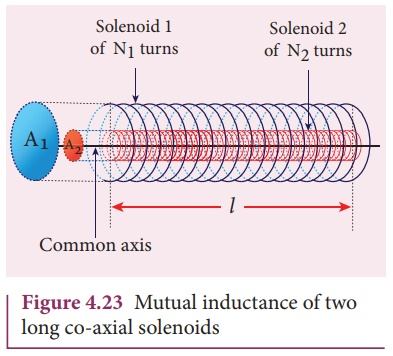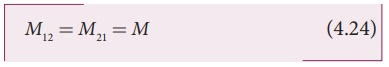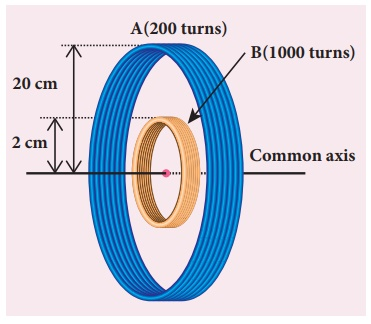Home | | Physics 12th Std | Mutual inductance between two long co-axial solenoids

# Mutual inductance between two long co-axial solenoids

Consider two long co-axial solenoids of same length l.

Mutual inductance between two long co-axial solenoids

Consider two long co-axial solenoids of same length l. The length of these solenoids is large when compared to their radii so that the magnetic field produced inside the solenoids is uniform and the fringing effect at the ends may be ignored. Let A1 and A2 be the area of cross section of the solenoids with A1 being greater than A2 as shown in Figure 4.23. The turn density of these solenoids are n1 and n2 respectively.Let i1 be the current flowing through solenoid 1, then the magnetic field produced inside it isAs the field lines of1 are passing through the area bounded by solenoid 2, the magnetic flux is linked with each turn of solenoid 2 due to solenoid 1 and is given byThe flux linkage of solenoid 2 with total turns N2 isComparing the equations (4.20) and (4.21),This gives the expression for mutual inductance M21 of the solenoid 2 with respect to solenoid 1. Similarly, we can find mutual inductance M12 of solenoid 1 with respect to solenoid 2 as given below.

The magnetic field produced by the solenoid 2 when carrying a current i2 is

B2 = ┬Ą n2i2

This magnetic field B2 is uniform inside the solenoid 2 but outside the solenoid 2, it is almost zero. Therefore for solenoid 1, the area A2 is the effective area over which the magnetic field B2 is present; not area A1. Then the magnetic flux ╬”12 linked with each turn of solenoid 1 due to solenoid 2 isThe flux linkage of solenoid 1 with total turns N1 isFrom equation (4.22) and (4.23), we can writeIn general, the mutual inductance between two long co-axial solenoids is given byIf a dielectric medium of relative permeability ┬Ąr is present inside the solenoids, then

M  = ┬Ą n1 n2 A2 l

(or) M = ┬Ą ┬Ąr n1 n2 A2 l

EXAMPLE 4.12

The current flowing in the first coil changes from 2 A to 10 A in 0.4 sec. Find the mutual inductance between two coils if an emf of 60 mV is induced in the second coil. Also determine the induced emf in the second coil if the current in the first coil is changed from 4 A to 16 A in 0.03 sec. Consider only the magnitude of induced emf.

Solution

Case (i):

di1 = 10 ŌĆō 2 = 8 A; dt = 0.4 s;

╬Ą2 = 60 ├Ś10ŌłÆ3V

Case (ii):

di1 = 16 ŌĆō 4 = 12 A;

dt = 0.03 s

(i) Mutual inductance of the second coil with respect to the first coil(ii) Induced emf in the second coil due to the rate of change of current in the first coil isEXAMPLE 4.13

Consider two coplanar, co-axial circular coils A and B as shown in figure. The radius of coil A is 20 cm while that of coil B is 2 cm. The number of turns is 200 and 1000 for coils A and B respectively. Calculate the mutual inductance of coil B with respect to coil A. If the current in coil A changes from 2 A to 6 A in 0.04 sec, determine the induced emf in coil B and the rate of change of flux through the coil B at that instant.Solution

NA = 200 turns; NB = 1000 turns;

rA = 20 ├Ś 10-2 m; rB = 2 ├Ś 10-2 m;

dt = 0.04 s; diA = 6ŌłÆ2 = 4A

Let iA be the current flowing in coil A, then the magnetic field BA at the centre of the circular coil A isThe rate of change of magnetic flux of coil isTags : Definition, Explanation, Formulas, Solved Example Problems | Electromagnetic Induction , 12th Physics : Electromagnetic Induction and Alternating Current
Study Material, Lecturing Notes, Assignment, Reference, Wiki description explanation, brief detail
12th Physics : Electromagnetic Induction and Alternating Current : Mutual inductance between two long co-axial solenoids | Definition, Explanation, Formulas, Solved Example Problems | Electromagnetic Induction